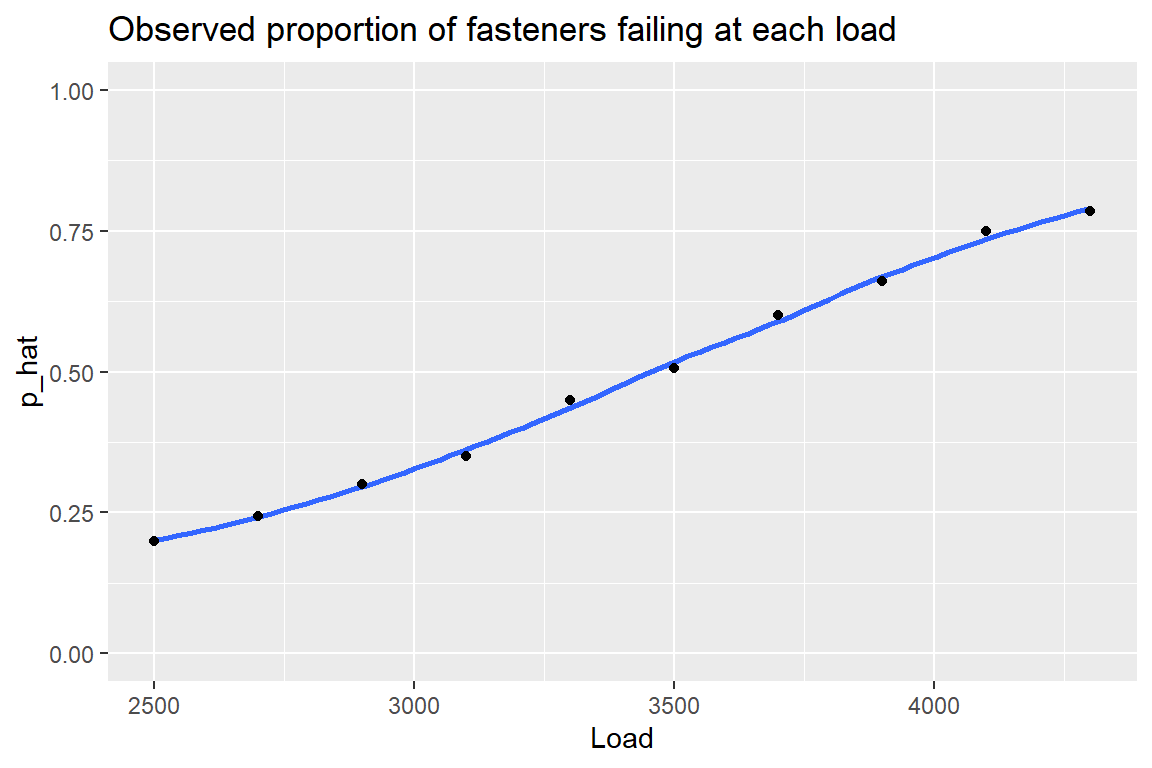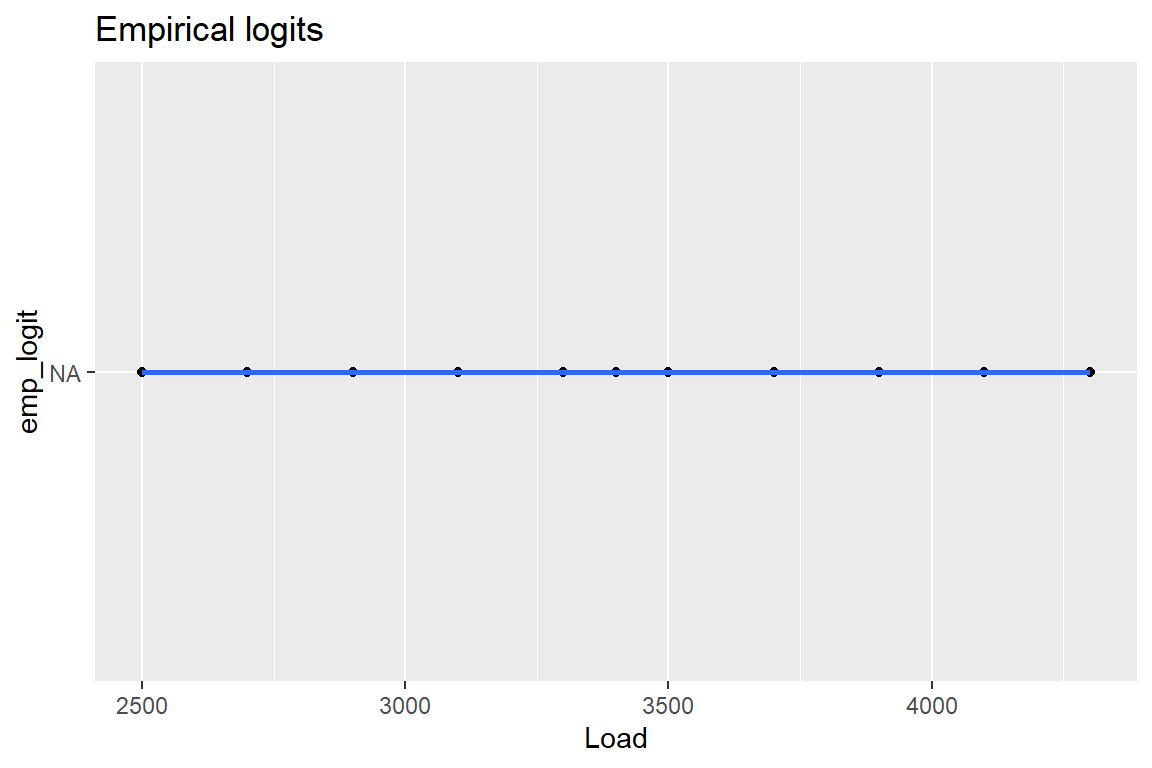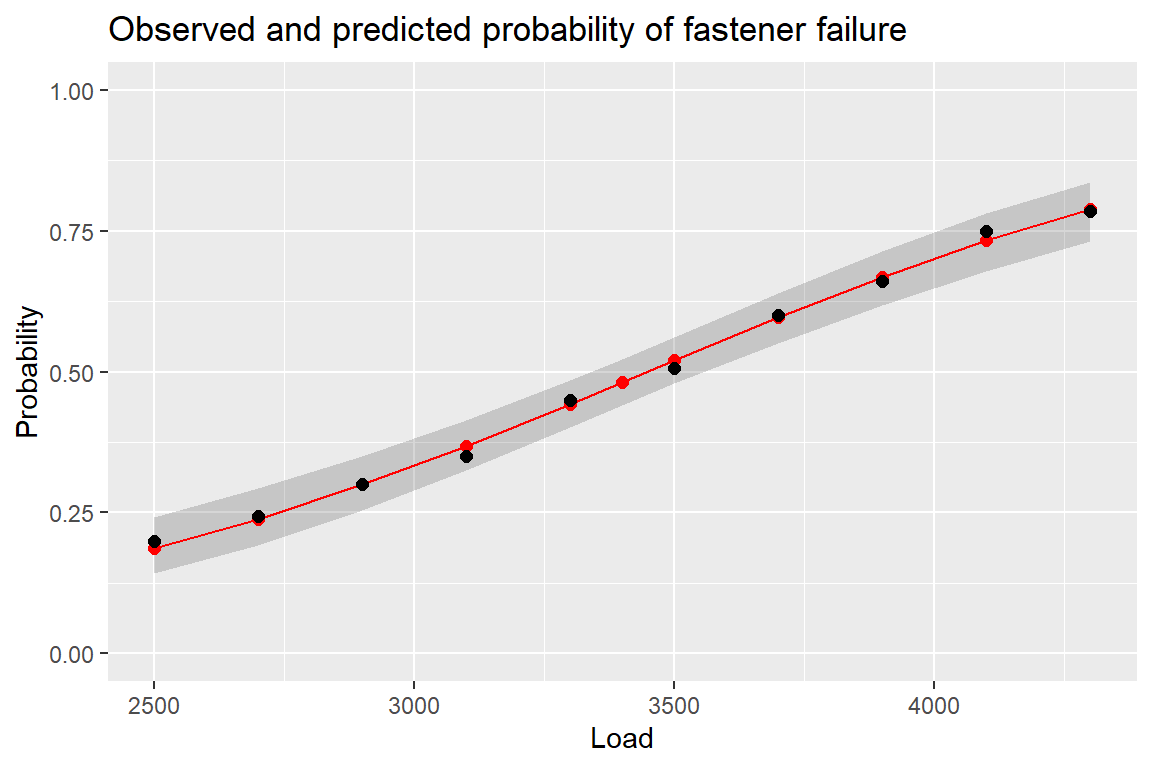# 1 Alloy fastener failures

The following data are from a study on the compressive strength of an alloy fastener used in the construction of aircraft. Ten pressure loads, increasing in units of 200 psi from 2500 psi to 4300 psi, were used with different numbers of fasteners being tested at each of the loads. The table below gives the number of fasteners failing out of the number tested at each load.

library(tidyverse)

dat_fastener <-
) %>%
# Augment the dataset with the Load we wish to predict in a later part.
bind_rows(
c(Load = 3400, Tested = NA, Failed = NA)
)

# view data
dat_fastener
# A tibble: 11 x 3
<dbl>  <dbl>  <dbl>
1  2500     50     10
2  2700     70     17
3  2900    100     30
4  3100     60     21
5  3300     40     18
6  3500     85     43
7  3700     90     54
8  3900     50     33
9  4100     80     60
10  4300     65     51
11  3400     NA     NA

## 1.1(1 p) Interpret plot of observed proportions against load

Compute the observed proportion of fasteners failing at each load.

# observed proportions
dat_fastener <-
dat_fastener %>%
mutate(
p_hat = Failed / Tested
)
library(ggplot2)
p <- ggplot(dat_fastener, aes(x = Load, y = p_hat))
p <- p + geom_smooth(se = FALSE)
p <- p + geom_point()
p <- p + scale_y_continuous(limits = c(0,1))
p <- p + labs(title = "Observed proportion of fasteners failing at each load")
print(p)Comment on how the proportion of failures depends on load.

## 1.2(2 p) Compute the empirical logits and interpret plot against load

Compute the empirical logits at each load.

# emperical logits
## replace "NA" with the equation for the empirical logits
## SOLUTION HERE
# emperical logits
dat_fastener <-
dat_fastener %>%
mutate(
emp_logit = NA
)

Present a graphical summary that provides information on the adequacy of a logistic regression model relating the probability of fastener failure as a function of load.

library(ggplot2)
p <- ggplot(dat_fastener, aes(x = Load, y = emp_logit))
p <- p + geom_point()
p <- p + geom_smooth(method = lm, se = FALSE)
p <- p + labs(title = "Empirical logits")
print(p)Interpret the plot regarding whether the empirical logits appear linear.

## 1.3(2 p) Fit a logistic regression model, interpret deviance lack-of-fit

Fit a logistic model relating the probability of fastener failure to load.

glm_fa <-
glm(
cbind(Failed, Tested - Failed) ~ Load
, family = binomial
, data = dat_fastener
)

# Test residual deviance for lack-of-fit (if > 0.10, little-to-no lack-of-fit)
dev_p_val <- 1 - pchisq(glm_fa$deviance, glm_fa$df.residual)
dev_p_val
 0.999957

Look at the residual deviance lack-of-fit statistic. Is there evidence of any gross deficiencies with the model?

## 1.4(2 p) Interpret logistic regression coefficients

Does load appear to be a useful predictor of the probability of failure? Interpret the hypothesis test.

summary(glm_fa)

Call:
glm(formula = cbind(Failed, Tested - Failed) ~ Load, family = binomial,
data = dat_fastener)

Deviance Residuals:
Min        1Q    Median        3Q       Max
-0.29475  -0.11129   0.04162   0.08847   0.35016

Coefficients:
Estimate Std. Error z value Pr(>|z|)
(Intercept) -5.3397115  0.5456932  -9.785   <2e-16 ***
Load         0.0015484  0.0001575   9.829   <2e-16 ***
---
Signif. codes:  0 '***' 0.001 '**' 0.01 '*' 0.05 '.' 0.1 ' ' 1

(Dispersion parameter for binomial family taken to be 1)

Null deviance: 112.83207  on 9  degrees of freedom
Residual deviance:   0.37192  on 8  degrees of freedom
(1 observation deleted due to missingness)
AIC: 49.088

Number of Fisher Scoring iterations: 3

## 1.5(1 p) Write model equation

Provide an equation relating the fitted probability of fastener failure to the load on the probability scale: $$\tilde{p} = \ldots$$. I have provided the equation on the logit scale.

The MLE of the predicted probabilities satisfy the logit equation $\log \left( \frac{\tilde{p}}{1-\tilde{p}} \right) = -5.34 + 0.00155 \textrm{ Load} .$

## 1.6(0 p) Plot the fitted probabilities as a function of Load

# predict() uses all the Load values in dataset, including appended values
fit_logit_pred <-
predict(
glm_fa
, data.frame(Load = dat_fastener$Load) , type = "link" , se.fit = TRUE ) %>% as_tibble() # put the fitted values in the data.frame dat_fastener <- dat_fastener %>% mutate( fit_logit = fit_logit_pred$fit
, fit_logit_se = fit_logit_pred\$se.fit
, fit_p        = exp(fit_logit) / (1 + exp(fit_logit))
# CI for p fitted values
, fit_p_lower  = exp(fit_logit - 1.96 * fit_logit_se) / (1 + exp(fit_logit - 1.96 * fit_logit_se))
, fit_p_upper  = exp(fit_logit + 1.96 * fit_logit_se) / (1 + exp(fit_logit + 1.96 * fit_logit_se))
)
library(ggplot2)
p <- ggplot(dat_fastener, aes(x = Load, y = p_hat))
# predicted curve and point-wise 95% CI
p <- p + geom_ribbon(aes(x = Load, ymin = fit_p_lower, ymax = fit_p_upper), alpha = 0.2)
p <- p + geom_line(aes(x = Load, y = fit_p), colour = "red")
# fitted values
p <- p + geom_point(aes(y = fit_p), size = 2, colour = "red")
# observed values
p <- p + geom_point(size = 2)
p <- p + scale_y_continuous(limits = c(0, 1))
p <- p + labs(title = "Observed and predicted probability of fastener failure"
, y = "Probability"
)
print(p)## 1.7(2 p) Interpret the prediction with 95% CI at 3400 psi

Compute the estimated probability of failure when the load is 3400 psi. Provide and interpret the 95% CI for this probability.

We have already augmented the data set with the 3400 psi value, so the predict() function above has already done the calculations for us.